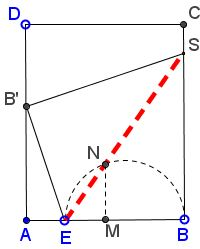# The Shortest Crease

### What this might be about?

18 October 2015, Created with GeoGebra

### Problem

An interesting optimization problem has been offered by Henry Ernest Dudeney:

Fold a page, so that the bottom outside corner touches the inside edge and the crease is the shortest possible. That is about as simple a question as we could put, but it will puzzle a good many readers to discover just where to make the fold. I give two examples of folding:It will be seen that the crease on the right is longer than that on the left, but the latter is not the shortest possible.

References

1. H. E. Dudeney, 536 Puzzles & Curious Problems, Charles Scribner's Sons, 1967

Bisect $AB$ in $M.$ Bisect $AM$ at $E.$ Draw the line at $M$ perpendicular to $AB$ and the semicircle with diameter $BE.$ Let $N$ be the intersection of the two.The line $EN$ gives the direction of the shortest possible crease under the conditions.

### Solution

The solution below is straightforward application of the Pythagorean theorem and the very beginning of the calculus.

Taking into account that Dudeney "folds a page," assume $AD\gt AB=4.$ Denote $x=BE.$Then successively: $AE=4-x;$ $EB'=BE=x;$ $AB'=\sqrt{x^{2}-(4-x)^{2}}.$

Further, triangles $AEB'$ and $FSB'$ are similar so that $\displaystyle FS=\frac{AE\cdot B'F}{AB'}=\frac{4(4-x)}{\sqrt{x^{2}-(4-x)^{2}}};$

\begin{align} BS&=BF+FS\\ &=\sqrt{x^{2}-(4-x)^{2}}+\frac{4(4-x)}{\sqrt{x^{2}-(4-x)^{2}}}\\ &=\frac{x^{2}-(4-x)^{2}+4(4-x)}{\sqrt{x^{2}-(4-x)^{2}}}\\ &=\frac{4x}{\sqrt{x^{2}-(4-x)^{2}}}\\ &=\frac{2x}{\sqrt{2(x-2)}}. \end{align}

Finally

\begin{align} ES^{2}&=BE^2+BS^2\\ &=x^{2}+\frac{4x^{2}}{2(x-2)}\\ &=\frac{x^{3}}{x-2}\\ \end{align}

Consider the function $\displaystyle f(x)=\frac{x^{3}}{\sqrt{x-2}}.$

$\displaystyle f'(x)=\frac{3x^{2}(x-2)-x^{3}}{(x-2)^{2}}=\frac{2x^{2}(x-3)}{(x-2)^2}.$

$x=0$ leads to no crease, but $x=3$ does. We next find that $f''(3)\gt 0$ so that $x=3$ is a local minimum.

This shows why Dudeney's answer is correct. With $x=3,$ $MN=\sqrt{2}$ and $BS=3\sqrt{2},$ implying that $ES$ passes through $N.$ Thus Dudeney's answer is a byproduct of the calculus solution. It would be exciting to arrive at his result without calculus.

Thus, according to Dudeney the minimum crease of a page with base $4$ equals $3\sqrt{3}.$ This crease can be obtained for any page whose vertical size is at least $3\sqrt{2}.$ However, it is obvious that, with the vertical side $b\lt 3\sqrt{3},$ the vertical crease through the midpoint $M$ will be shorter than $3\sqrt{3}.$ Obviously, Dudeney assumed that, for a "page," the ratio of the vertical to the horizontal side exceeds $3\sqrt{3}/4.$

Paper Folding Geometry### A Sample of Optimization Problems III

• Mathematicians Like to Optimize
• Mathematics in Pizzeria
• The Distance to Look Your Best
• Building a Bridge
• Linear Programming
• Residence at an Optimal Distance
• Distance Between Projections
• Huygens' Problem
• Optimization in a Crooked Trapezoid
• Greatest Difference in Arithmetic Progression
• Area Optimization in Trapezoid
• Minimum under Two Constraints
• Optimization with Many Variables
• Minimum of a Cyclic Sum with Logarithms
• A Problem with a Magical Solution from Secrets in Inequalities
• Leo Giugiuc's Optimization with Constraint
• Problem 4033 from Crux Mathematicorum
• An Unusual Problem by Leo Giugiuc
• A Cyclic Inequality With Constraint in Two Triples of Variables
• Two Problems by Kunihiko Chikaya
• An Inequality and Its Modifications
• A 2-Variable Optimization From a China Competition
•